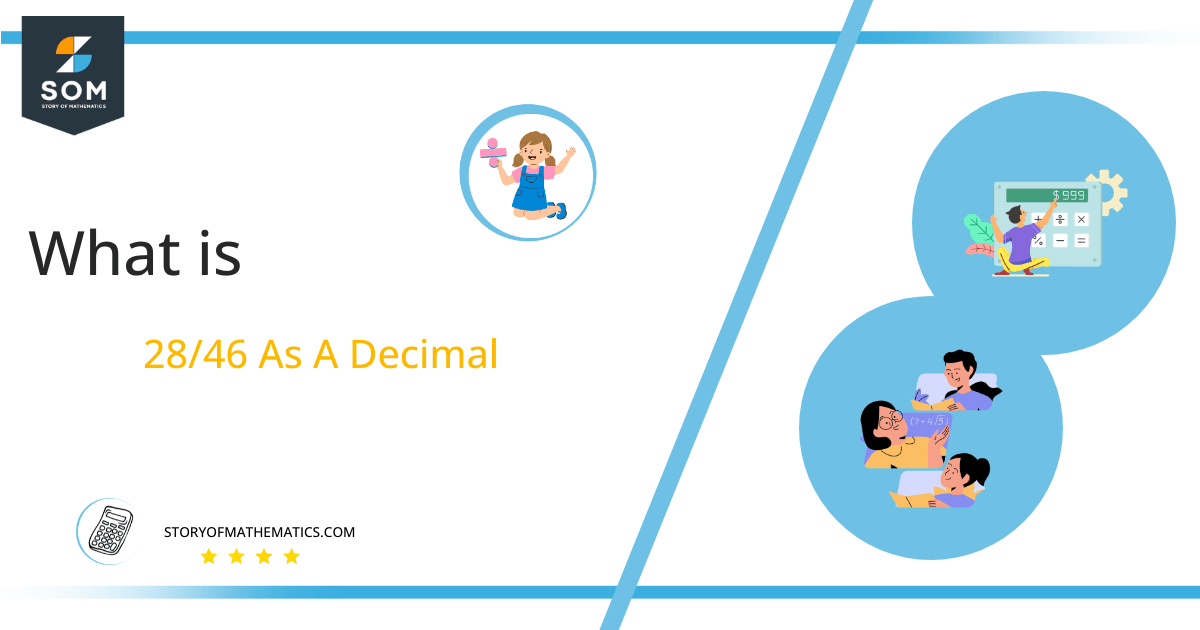# What Is 28/46 as a Decimal + Solution With Free Steps

The fraction 28/46 as a decimal is equal to 0.608695652.

When converting Fractional quantities to Decimal values, the division operator is needed. The p/q form, where p and q are referred to as the Numerator and Denominator, can be used to represent a Fraction.Here, we are more interested in the division types that result in a Decimal value, as this can be expressed as a Fraction. We see fractions as a way of showing two numbers having the operation of Division between them that result in a value that lies between two Integers.

Now, we introduce the method used to solve said fraction to decimal conversion, called Long Division, which we will discuss in detail moving forward. So, let’s go through the Solution of fraction 28/46.

## Solution

First, we convert the fraction components, i.e., the numerator and the denominator, and transform them into the division constituents, i.e., the Dividend and the Divisor, respectively.

This can be done as follows:

Dividend = 28

Divisor = 46

Now, we introduce the most important quantity in our division process: the Quotient. The value represents the Solution to our division and can be expressed as having the following relationship with the Division constituents:

Quotient = Dividend $\div$ Divisor = 28 $\div$ 46

This is when we go through the Long Division solution to our problem.Figure 1

## 28/46 Long Division Method

We start solving a problem using the Long Division Method by first taking apart the division’s components and comparing them. As we have 28 and 46, we can see how 28 is Smaller than 46, and to solve this division, we require that 28 be Bigger than 46.

This is done by multiplying the dividend by 10 and checking whether it is bigger than the divisor or not. If so, we calculate the Multiple of the divisor closest to the dividend and subtract it from the Dividend. This produces the Remainder, which we then use as the dividend later.

Now, we begin solving for our dividend 28, which after getting multiplied by 10 becomes 280.

We take this 280 and divide it by 46; this can be done as follows:

280 $\div$ 46 $\approx$ 6

Where:

46 x 6 = 276

This will lead to the generation of a Remainder equal to 280 – 276 = 4. Now this means we have to repeat the process by Converting the 4 into 40.

Still, the dividend is less than the divisor, so we will multiply it by 10 again. For that, we have to add the zero in the quotient. So, by multiplying the dividend by 10 twice in the same step and by adding zero after the decimal point in the quotient, we now have a dividend of 400.

400 $\div$ 46 $\approx$ 8

Where:

46 x 8 = 368

This, therefore, produces another Remainder which is equal to 400 – 368 = 32.

Finally, we have a Quotient generated after combining the two pieces of it as 0.608=z, with a Remainder equal to 32.Images/mathematical drawings are created with GeoGebra.